# Power Factor Correction (PFC)Get valuable resources straight to your inbox - sent out once per month

Power factor is defined as the ratio of energy a device is capable of transmitting to the output versus the total amount of energy it takes from the input power source. It is a key figure of merit for the design of electrical devices, especially due to the regulations put in place by countries and international organizations like the EU, which define the minimum power factor or maximum level of harmonics a device must have in order to be sold in the European market.

The reason why these organizations are so invested in improving power factor is because low-quality power is a real threat to the power grid, increasing heating losses and potentially causing a power failure.

There are two main causes of poor power factor:

• Displacement: This occurs when a circuit’s voltage and current waves are out of phase, usually due to the presence of reactive elements such as inductors or capacitors.
• Distortion: Defined as the alteration of the wave’s original shape, this is usually caused by nonlinear circuits, such as rectifiers. These nonlinear waves have a lot of harmonic content, which distorts the voltage in the grid.

Power factor correction (PFC) is the series of methods used to try to improve a device’s power factor.

In order to fix displacement issues, external reactive components are commonly used to compensate the circuit’s total reactive power.

To solve distortion problems, there are two options:

• Passive power factor correction (PFC): Improves PF by filtering out harmonics using passive filters. This is typically used in low-power applications, but is not enough at high power.
• Active power factor correction (PFC): Uses a switching converter to modulate the distorted wave in order to shape it into a sine wave. The only harmonics present in the new signal are at the switching frequency, so they are easily filtered out. This is considered the best PFC method, but adds complexity to the design.

A good power factor correction circuit is a crucial element for any modern design, because a device with a bad power factor is going to be inefficient, will put an unnecessary strain on the grid, and possibly cause problems to the rest of connected devices.

## The Need for Power Factor Correction (PFC) in AC/DC Power Supplies

As discussed in our previous article, an AC/DC power supply is made up of several circuits that transform an input AC voltage into a stable DC voltage at the output. The most essential of these circuits is the rectifier, which is responsible for transforming the AC voltage into a DC voltage; however, this circuit alone is not enough to ensure adequate operation.

In order for an AC/DC power supply to be efficient and safe, it needs to incorporate isolation, power factor correction (PFC), and voltage reduction. These elements protect the user, the grid, and any connected devices, and are each integrated to some extent in all switching power supplies.

The first step in any switching power supply is the rectification of the input voltage. Rectification is the process of converting a signal from AC to DC, and is done using a rectifier. The negative voltage in the AC wave can be either cut off using a half-wave rectifier, or inverted using a full-wave rectifier.

A full-wave rectifier is made up of four diodes connected in an arrangement called a Graetz bridge. These diodes switch on and off as the voltage from the power supply goes from negative to positive, inverting the negative half-wave’s polarity and turning the AC sine wave into a DC wave (see Figure 1).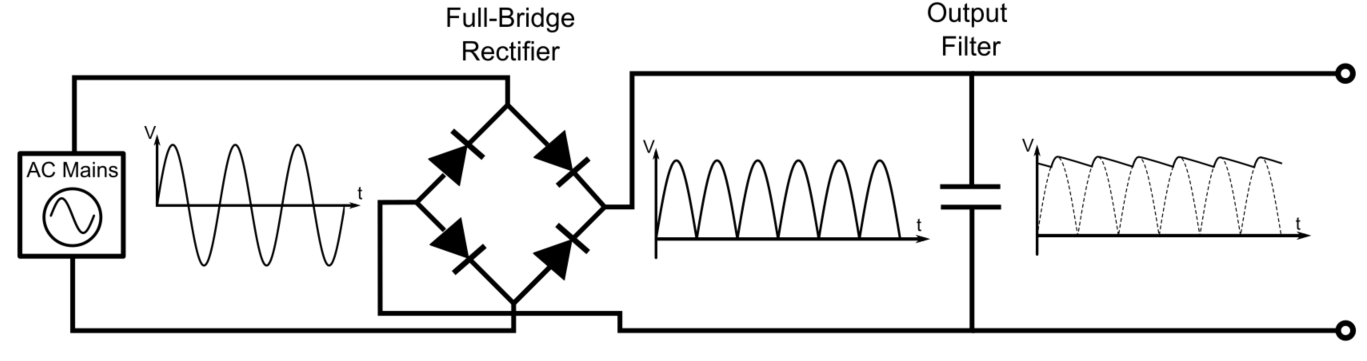Figure 1: Schematic of a Full-Bridge Rectifier

This wave has a large voltage variation, called ripple voltage, so a reservoir capacitor is connected in parallel to the diode bridge to help smooth the output voltage ripple.

However, if you observe the waveform of the rectifier’s reservoir capacitor, you’ll see that the capacitor is charged during a very short time, from the point where the voltage at the input of the capacitor is greater than the capacitor’s charge, to the rectified signal’s peak. This creates a series of short current spikes in the capacitor, which look nothing like a sinusoid (see Figure 2).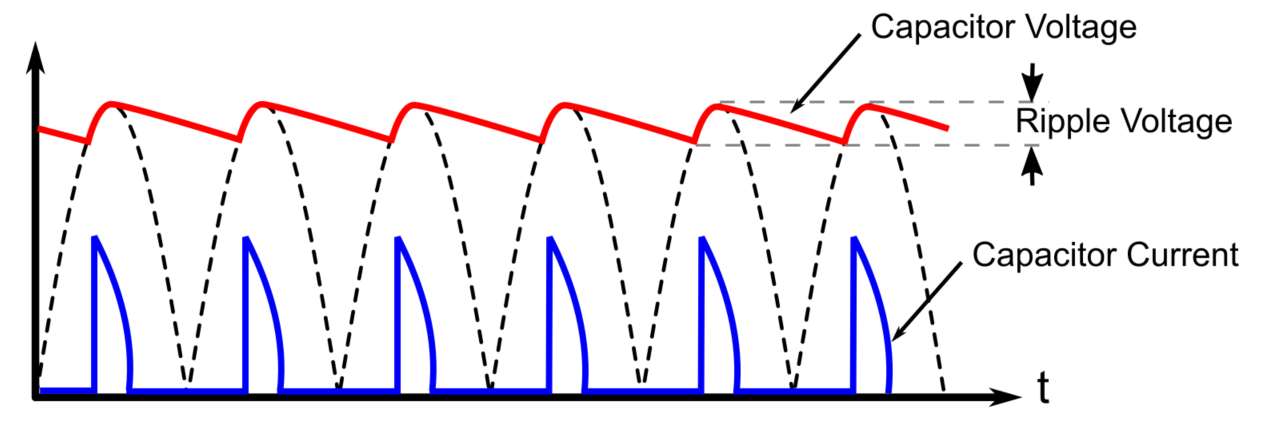Figure 2: Voltage and Current Waveforms at the Rectifier Output

This is a very big problem, not only for the power supply, but for the entire power grid. To understand the magnitude of this problem, we must first have a handle on the concept of harmonics.

## Harmonics and the Fourier Transform

Most of the electric waveforms you have seen up to now are sine waves. However, waves often stop being purely sinusoidal, especially when reactive components (capacitors, inductors) or nonlinear components (transistors, diodes) are present. These waves are defined by different, often complex mathematical functions. This could make the analysis of this wave much more difficult, because the mathematics behind the analysis become considerably harder (see Figure 3).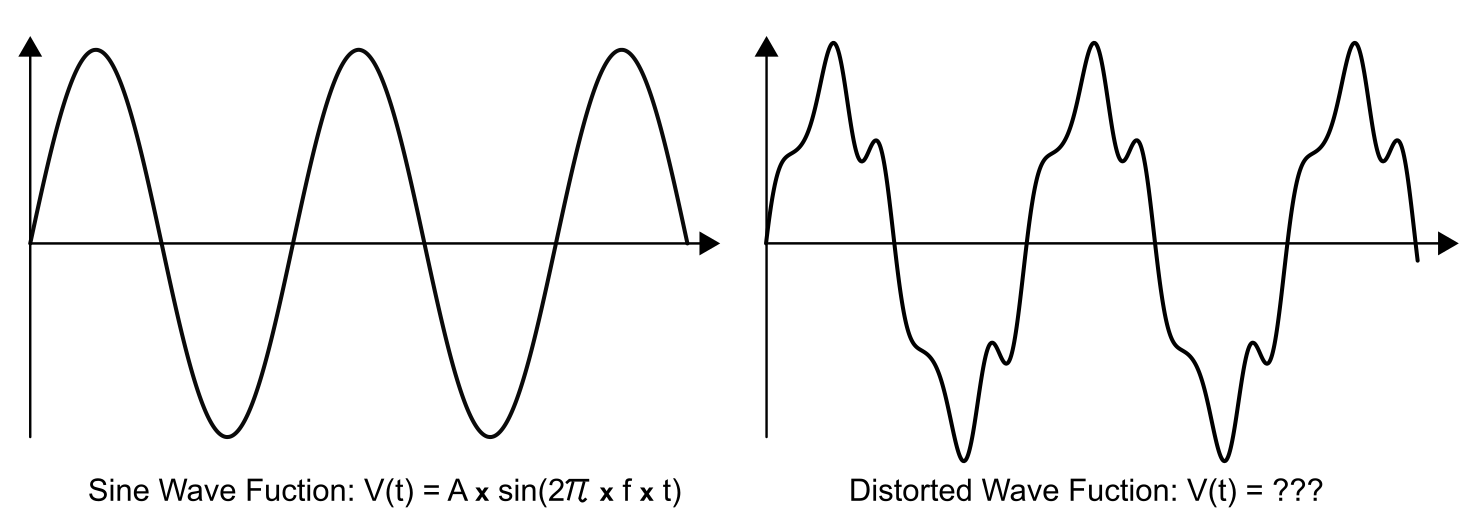Figure 3: Sinusoid vs. Distorted Wave – Waveform and Wave Function

Fortunately, in the 19th century, French mathematician Jean-Baptiste Joseph Fourier came up with a method of decomposing any arbitrary periodic waveform into a series of sine and cosine waves with different frequencies, called harmonics (see Figure 4). The first of these waves, which is referred to as the fundamental, is the wave with the lowest frequency. Then, several other waves are combined with the fundamental and given specific amplitudes and frequency. As a rule of thumb, the more that a waveform’s shape deviates from a pure sinusoid, the more harmonics it will have.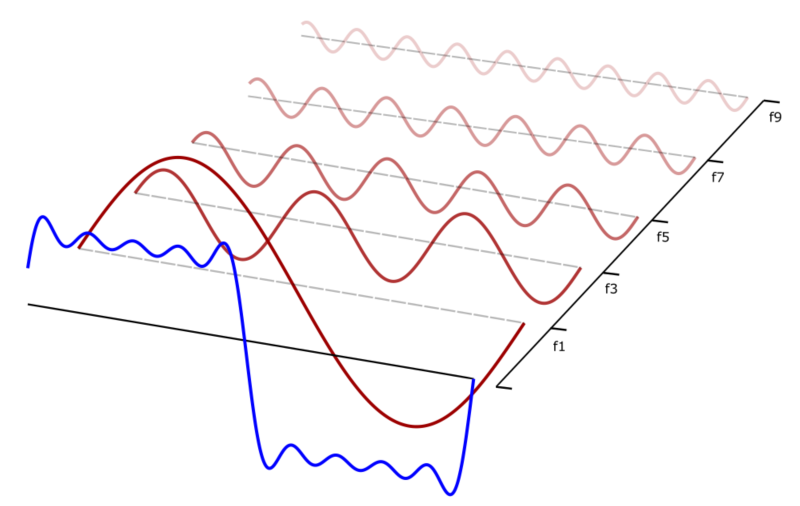Figure 4: Decomposition of an Arbitrary Waveform into a Fourier Series

Harmonic frequencies must be integer multiples of the fundamental frequency. For example, if a wave’s fundamental frequency is 50Hz, the second harmonic frequency will be 100Hz, the third will be 150Hz, and so on.

One of the most important parameters of harmonics is their amplitude, which is a measure of their effect on the fundamental frequency. Usually, the fundamental frequency has the largest amplitude, and the harmonic’s amplitude decreases proportionately to its order, so the 9th or 20th harmonic will be practically nonexistent. The amplitude of these harmonics can be graphed, showing what role each harmonic plays in the creation of the arbitrary waveform.

The problem with the current in the capacitor is that it looks very similar to a delta function. This type of wave is, ideally, an infinitely short, infinitely powerful pulse. A wave of this shape is understandably complicated to decompose into sine waves, and results in a large number of powerful harmonics, spanning practically all frequencies (see Figure 5).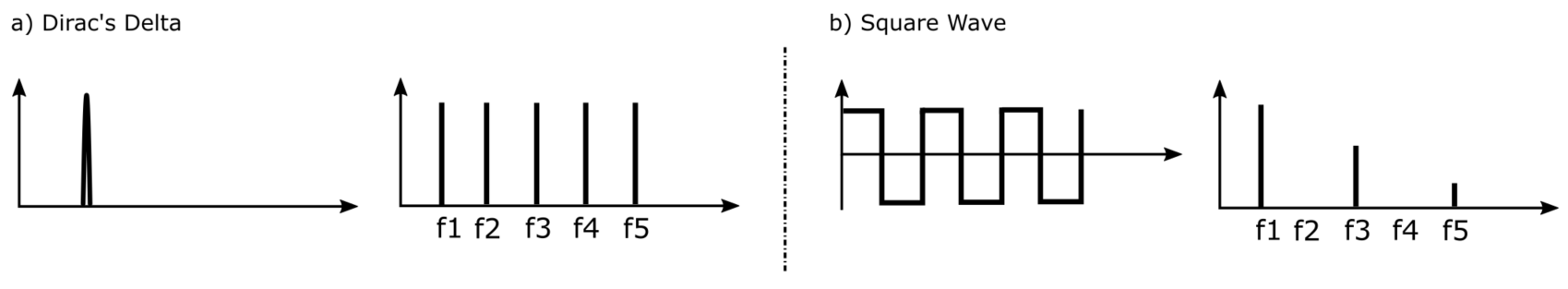Figure 5: Harmonic Distribution of a Delta Function and a Square Wave

This isn’t necessarily a problem, because the device still supplies power to the load, so many low-power AC/DC power supply manufacturers don’t do anything about it, because it only affects the power supply’s power factor. However, if there are too many high-power devices with low power factor connected to the grid, it could be problematic, and even cause a blackout!

## Power Factor

There are three types of AC power. The first is called active power, and is usually referred to as real power, or P. This represents the net energy that is transferred to a load. If the load is purely resistive, all power in the line is active power, and the voltage and current oscillate in phase with each other. Second, if the load is purely reactive, like an inductor or a capacitor, the power will be purely reactive, often expressed as Q. This power is used to generate and maintain the magnetic and electric fields in reactive components. These fields make the current slip out of phase relative to the voltage, 90° ahead for capacitive loads, and 90° behind for inductive ones (see Figure 6). This means that the overall power generated by these purely reactive loads is zero, because the positive reactive power is canceled out by the negative reactive power.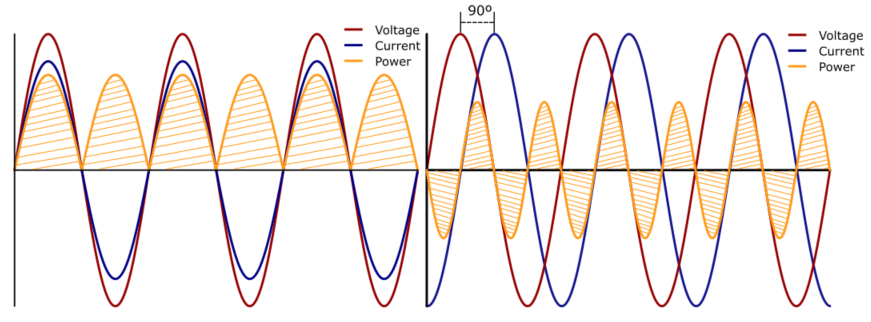Figure 6: In-Phase V-I Waves and Associated Power (Left). V-I Waveforms and Associated Power for a 90° Phase Difference (Right)

In practical applications, loads are never purely resistive or reactive, but are a combination of both. The third AC power type is the addition of both active and reactive power, and is called apparent power, or S. This addition is quadratic, and the relationship between active, reactive, and apparent power is often expressed in the form of a triangle.

Power factor is the relation between the active power and the apparent power, and is useful for measuring the efficiency of power transmission in the circuit (see Figure 7).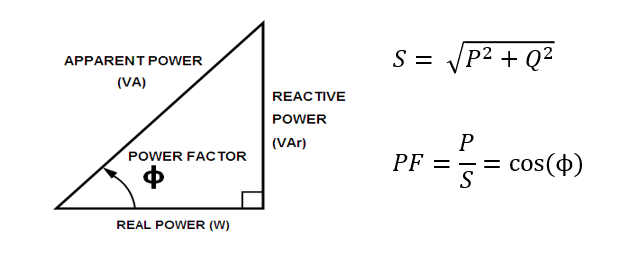Power Triangle

A low power factor is a combination of two factors: displacement and distortion. The first, in linear loads, is due to the presence of reactive components that make the current and voltage waves fall out of phase. The effect that the phase difference between the voltage and current has on the total power factor is defined by the displacement factor, which is calculated as the cosine of the angle between waves using Equation (1):

$$PF_{DISPLACEMENT}= COS (\theta_{V}-\theta_{I})$$

However, if we return to the matter at hand, the problem faced by power supply designers is not only that the current and the voltage waves may be out of phase, but also the fact that the current waveform has become a pulse train, which is a nonlinear function. This means that the multiplication of the voltage and current, also known as power, is also nonlinear and highly inefficient. This occurs when a circuit has nonlinear loads, such as fluorescent lights, electronic devices, and full-bridge rectifiers. These loads draw current in very short and abrupt bursts, which generates a very large quantity of harmonics, adding distortion to the signal. The most frequent way of describing the amount of distortion present in a signal due to the presence of harmonics is through the magnitude of total harmonic distortion (THD), which represents the proportion of harmonic current relative to the fundamental current. THD can be calculated using Equation (2):

$$THD = \frac {\sqrt {\sum n ≠1 \space I_{n}^2 \space RMS}}{ {I_{fund. \space RMS}}} = \frac {RMS \space harmonic \space current}{RMS \space fundamental \space current}$$

The effect of distortion on the total power factor, however, is using the distortion factor, which is related to the total harmonic distortion with Equation (3):

$$PF_{DISTORTION}= \sqrt \frac {1}{1+THD^2}$$

The product of the displacement factor and the distortion factor makes up the power factor, calculated with Equation (4):

$$PF = PF_{DISPLACEMENT}\space x \space PF_{DISTORTION}= COS(\theta_{V}-\theta_{I}) \space x \space \sqrt \frac {1}{1+THD^2}$$

Power factor doesn’t often significantly affect a device’s operation, but when the current is pumped back into the grid, it brings the poor power factor with it. For example, if an inductive load with a very low power factor, such as the DC engine in a blender, is connected to the grid, the neighboring house’s TV screen may start to flicker because of the harmonics injected by the engine. At a large scale, this causes significant heating loss in the grid, and could even cause a power outage.

Understandably, power suppliers have put forward limitations into the amount of power interference a device can apply to the grid. The first attempt to do this was in 1899, with the start of electric lighting, when they realized that interference from other devices was making incandescent lights flicker. Then, in 1978, a regulation from the International Electrotechnical Commission (IEC) was put forward to force the introduction of power factor correction in consumer products.

Since then, different countries have created their own guidelines and regulations on power factor limitations. In the United States, the voluntary Energy Star guideline states that any computing equipment must have a PF of at least 0.9 when working at its maximum rated output. In the EU, the legislation (IEC31000-3-2) is more stringent, dividing electrical devices into four categories: appliances (A), power tools (B), lighting (C), and electronic devices (D). Each category has specific limitations on the relative weight that each harmonic (up to the 39th) can have relative to the fundamental frequency. Other countries have their own specific versions of this legislation, such as the Chinese GB/T 14549-93 or the international IEEE 519-1992.

Figure 8 shows the waveform restrictions established by the IEC61000-3-2 for class-C devices, both in the frequency domain and the time domain. As you can see, the maximum harmonic amplitude values in the frequency domain follow the shape of a square wave, which is confirmed by observing the resulting waves in the time domain.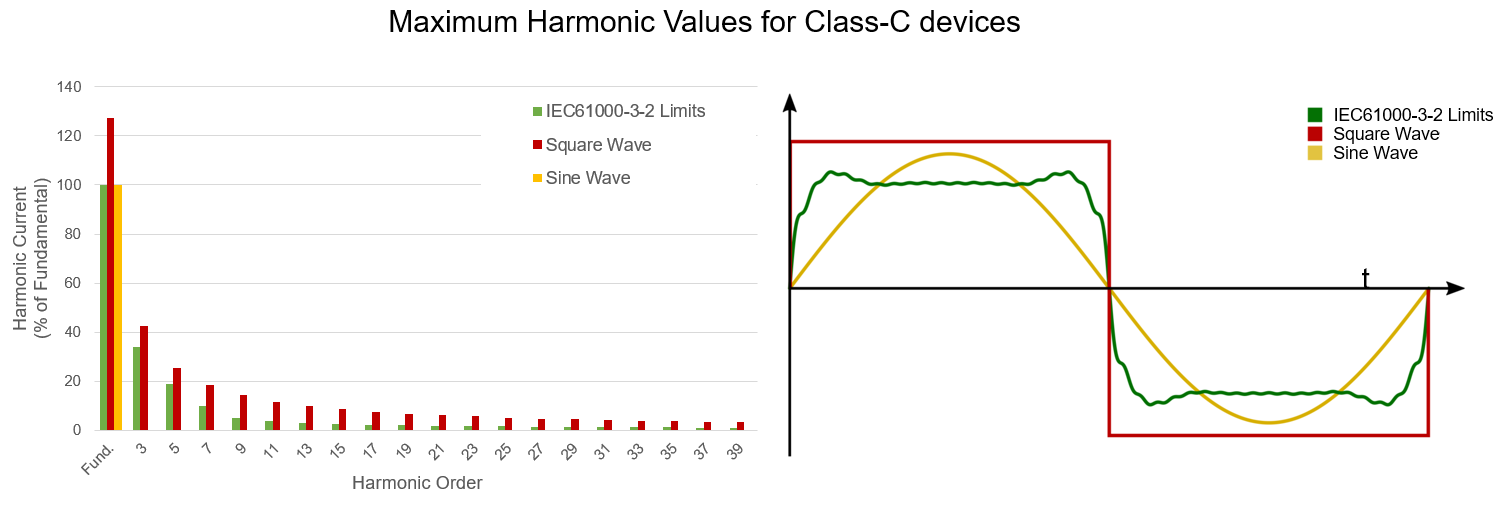Figure 8: Maximum Harmonic Values for Class-C devices in the Frequency (Left) and Time (Right) Domains

Although the waveform defined by IEC61000-3-2 looks very different from an ideal sine wave, it isn’t very difficult to find a device without PFC exceeding the regulated limits on harmonics and power factor. Therefore, a good power factor correction circuit is necessary in any commercialized device in order to increase operation efficiency and to be able to sell the device as a consumer product.

## What is Power Factor Correction (PFC)?

Power factor correction (PFC) is a series of methods that manufacturers of electronic devices use to improve their power factor.

As previously mentioned, a low power factor is caused by the presence of displacement or distortion in the signal. The negative effect of displacement on the power factor is relatively simple to solve, because capacitors drag the phase forward, while inductors drive it back. If a system’s current wave is lagging behind the voltage, you can simply add a capacitor with the right impedance to the circuit, and the current wave’s phase will be pulled forward until it is in phase with the voltage (see Figure 9).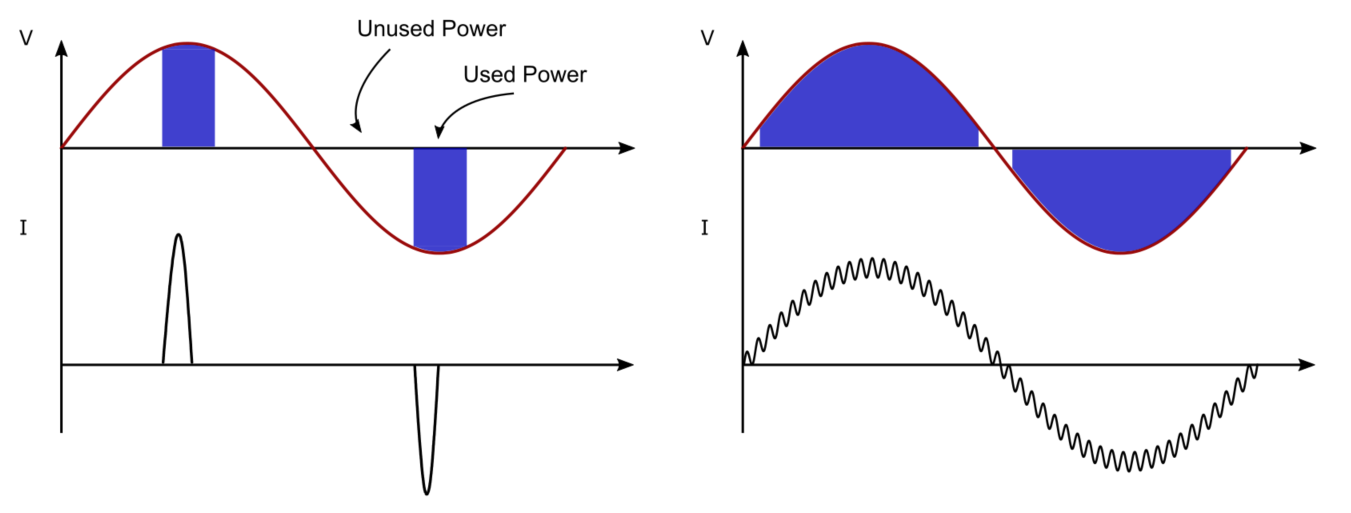Figure 9: Low PF Power Transmission with No PFC (Left) and Power Transmission with Corrected Power Factor and PFC (Right)

On the other hand, improving a system’s distortion factor, which is usually present in nonlinear circuits, is slightly more complicated than compensating the displacement factor found in linear circuits. To do so, there are two options:

1. Filter out harmonics: Accept the loss in efficiency, but try to reduce the number of harmonics injected into the grid by adding filters at the input. This is called passive PFC, and uses a low-pass filter aimed at eliminating the higher order harmonics, ideally leaving only the 50Hz fundamental frequency (see Figure 10). In practical applications, it is not very effective at improving a device’s PF, and is also impractical for high-power solutions, because of the loss of efficiency, size, and weight of the necessary capacitors and inductors. It isn’t typically used in applications with powers above hundreds of watts.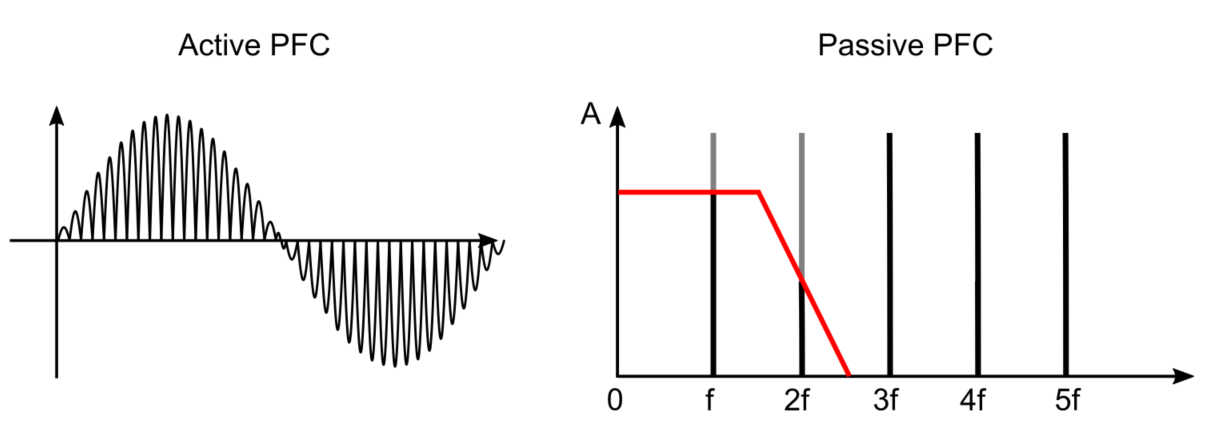Figure 10: Active PFC in DCM Mode, Output Current Waveform (Left) and Passive PFC Filter Frequency Response (Right)

1. Active power factor correction. This method changes the current waveform’s shape, making it follow the voltage. This way, the harmonics are moved to much higher frequencies, making them much easier to filter out. The most widely used circuit for these cases is a boost converter (see Figure 11). This circuit drives DC voltage up, while reducing its current, similar to a transformer. The simplest boost converter is composed of an inductor, a transistor, and a diode.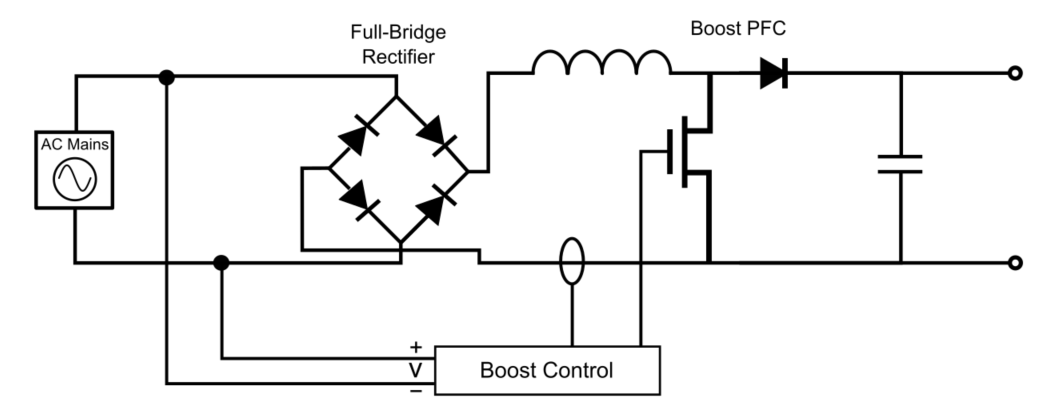Figure 11: Switched-Mode AC/DC Power Supply with Active PFC

The boost converter has two stages of operation. In the first stage, when the switch is closed, the inductor is charged by the voltage source (in this case, the voltage coming out of the rectifier). When the switch opens, the inductor injects the current it had stored in the previous stage into the circuit, increasing the voltage at the output. This current also charges a capacitor, which is responsible for maintaining the voltage level at the output while the inductor recharges.

If the switching frequency is high enough, neither the inductor nor the capacitor ever fully discharge, and the load at the output always has a greater voltage than the input voltage source. This is called continuous conduction mode (CCM). The longer the switch is closed (i.e. the longer the transistor is on), the larger the voltage at the output will be. If the duty cycle (the time the switch is on relative to the total switching cycle) is properly controlled, the input current wave can be shaped into a sinusoid.

However, not all PFC converters use CCM. There is another method that offers fewer switching losses and cheaper circuits, although it sacrifices final PF quality. This method, called boundary conduction mode (BCM) or critical conduction mode, switches the transistor just as the inductor is completely discharged (see Figure 12). This is called zero-current switching (ZCS), which allows the diode in the boost converter to change polarity faster and more easily, reducing the need for high-quality, expensive components.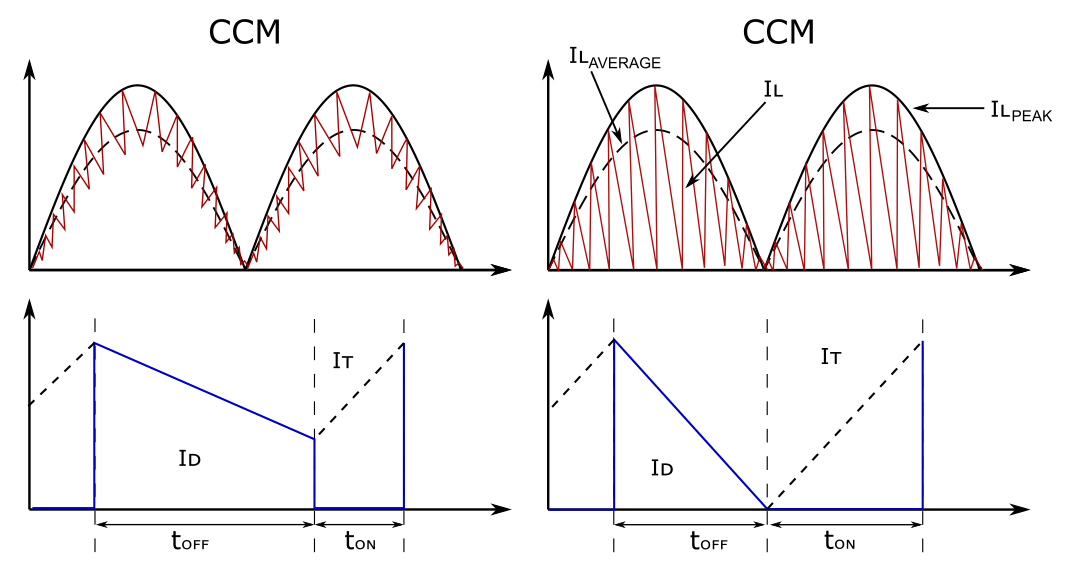Figure 12: PFC Inductor, Transistor, and Diode Current for Continuous Conduction Mode (Left) and Boundary Conduction Mode (Right)

The converter tracks the input voltage, so the output current looks like a sine wave with a frequency of 50Hz. However, this current waveform still looks very different from a pure sinusoid, so it will logically have a large number of harmonic components. Because these harmonic components are multiples of the switching frequency, which is much higher (50kHz to 100kHz) than the 50Hz fundamental, they will be very effectively filtered out. This significantly increases the power factor, which is why some switching power supplies reach PF values of up to 0.99.

One implementation of a BCM power factor corrector is the MP44010 controller. When connected to a boost converter, the ZCS pin detects when the inductor is discharged and activates the MOSFET (Q1 in Figure 13). This IC also compares the current and the voltage, shaping the current peaks to follow the shape of the input voltage.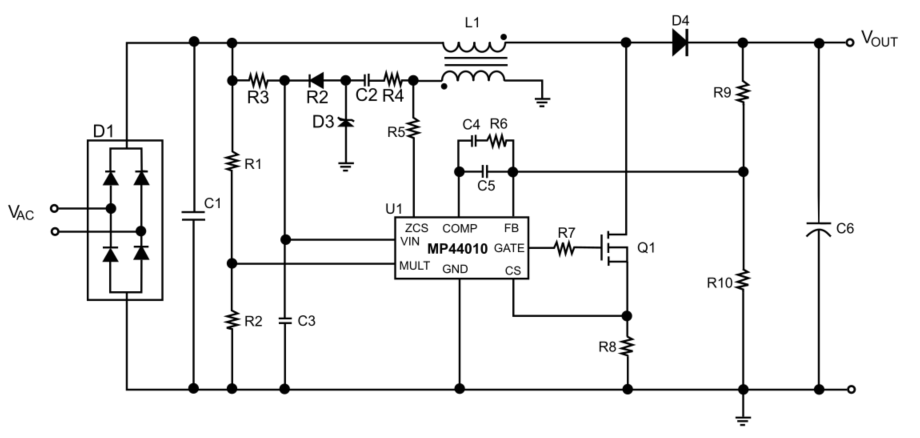Figure 13: MP44010 Typical Application Circuit

## Summary

Power factor is a key element to take into account in the design of any electronic device, but especially in the case of AC/DC power supplies. However, the selection of the right PFC circuit means analyzing the different tradeoffs available.

First, detect whether the origin of your low power factor is due to displacement or distortion. Then, depending on the amount of power in the circuit, select active or passive PFC. For active PFC, the designer will need to choose between implementing the converter in continuous or boundary conduction mode, which is a tradeoff between efficiency and power factor quality.

Whatever solution you decide to implement, MPS has a wide range of PFC controllers available, and our engineers are also ready to assist you with any questions you may have.

Did you find this interesting? Get valuable resources straight to your inbox - sent out once per month!

Get technical support